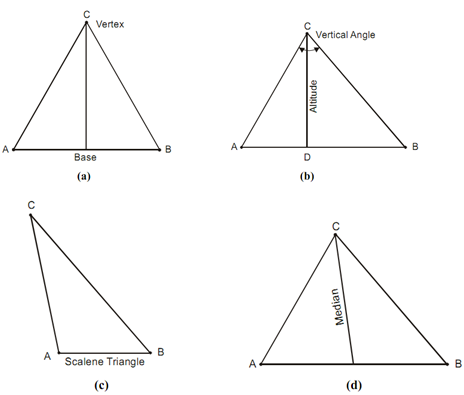## Triangle Assignment Help

Assignment Help: >> Geometrical Construction - Triangle

Triangle:

A triangle is a plane figure formed via three straight lines containing three angles. The side on that it is supposed to stand is called its base and angles at the base are recognized as the base angles. Such the point whereas the other two sides meet is called the vertex and the angle at the vertex is called the vertical angle in diagram (a). The line drawn from the vertex and perpendicular to the base or the base produced, if essential, is called the altitude in diagram (b).

(a) An equilateral triangle is that whether all the three sides are equal.

(b) An isosceles triangle is that whether two sides as well as the angles opposite to them are equivalent.

(c) A scalene triangle has none of its sides and angles equal. The altitude may either be within or outside the triangle as depicted in diagram (c).

(d) The line joining the angular point of a triangle to the middle point of the opposite side of the angular point is called the median as shown in diagram (d).#### Assured A++ Grade

Get guaranteed satisfaction & time on delivery in every assignment order you paid with us! We ensure premium quality solution document along with free turntin report!

All rights reserved! Copyrights ©2019-2020 ExpertsMind IT Educational Pvt Ltd